Goseeko blog# What is rank correlation?

## Overview(rank correlation)

A group of n individuals may be arranged in order to merit with respect to some characteristics. The same group would give different orders for different characteristics. Considering the orders corresponding to two characteristics A and B, the correction between these n pairs of rank is called the rank correlation in the characteristics A and B for that group of individuals.

## formula

Let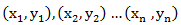be the ranks of  individuals corresponding to two characteristics.

Assuming nor two individuals are equal in either classification, each individual takes the values 1, 2, 3,  and hence their arithmetic means are, each

Suppose that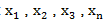be the values of variable X  and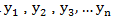those of Y.

Then

Here X and Y are deviations from the mean.

Which is called the rank correlation or Spearman’s rank correlation coefficient and we denote it by Rho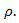## Solved examples

Example: Compute Spearman’s rank correlation coefficient r for the following data:

Solution:

Example. Three judges A,B,C give the following ranks. Find which pair of judges has common approach

A 1 6 5 10 3 2 4 9 7 8

B 3 5 8 4 7 10 2 1 6 9

C 6 4 9 8 1 2 3 10 5 7

Solution

Here n = ten, so that we get the following table,

.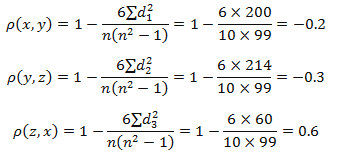Since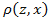is maximum, so that the pair of judge A and C have the nearest common approach.

Interested in learning about similar topics? Here are a few hand-picked blogs for you!